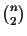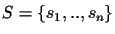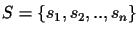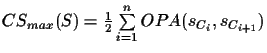Next: Scoring of MSAs without Up: Methods Previous: Circular tours

## Traveling Salesman Problem Application

The probability (exponential of the score) derived from pairwise alignments are now the key to identifying a circular tour. For a set of protein sequences it is computationally simple to obtain a set ofpairwise scores by aligning each sequence with every other sequence using a DP algorithm to obtain the Optimal Pairwise Alignment. We shall refer to these as OPA scores [Carillo and Lipman, 1988], to distinguish (see below) these from a pairwise alignment inferred from an MSA. Our goal is to be able to find a circular tour without the need of constructing an evolutionary tree. As we have shown above, a circular tour is the shortest possible tour for a tree (see Definition 2.2). Note that a shorter distance corresponds to a higher score. A non-circular tour has at least some edge of the tree traversed more than twice, and no edge less than twice. In this paper we have so far always been using some tree, which we don't have in reality. The only information available to us is just the sequences and the OPA scores. So suppose now we do not have any information about the tree for that given set of sequences. But we know that the tree has some circular tour. We also know that a circular tour is the shortest possible tour through that tree, and that the best tree has the shortest total path length (sum of all edges) of all possible trees, since we want the tree with the maximum probability (see also section 2.4.1).

Problem 2.1 (TSP problem)   Given is a set of sequencesand the corresponding scores of the optimal pairwise alignments. The problem is to find the longest tour where each sequence is visited once.

Definition 2.3   The TSP order TSP(S) of a set of sequencesis the order of the sequences that is derived from the optimal solution of a TSP.

Hence to find such a circular tour, we need to find the shortest tour from leaf to leaf of the given set of sequences S. To solve this problem we reduce it to the symmetric Traveling Salesman Problem (TSP): given is a matrix M that contains thedistances of n cities [Johnson, 1990,Johnson, 1987]. The problem is to find the shortest tour where each city is visited once. We use a modified version of the problem: in our case, the cities correspond to the sequences and the distances are the scores of the pairwise alignments. In addition, we are interested in the longest, not shortest tour, as our scores correspond to probabilities 1. The TSP is very well studied and optimal solutions can be calculated within a few hours for up to 1000 cities and in a few seconds for up to 100 cities. There are heuristics for large scale problems that calculate near optimal solutions that are within 1% to 2% of the optimum [Padberg and Rinaldi, 1991,Groetschel and Holland, 1991]. For real applications we have seldom more than 100 sequences to compare simultaneously, and the calculation of the optimal TSP solution usually takes only a small fraction of the time it takes to compute all pairwise alignments to derive the scores. We refer to the sum of OPA scores in circular order C(S) as CSmax(S).

Definition 2.4   Let C(S) be the output permutation of a TSP algorithm, thenfor a given set of sequencesis:, where Cn+1 = C1.Next: Scoring of MSAs without Up: Methods Previous: Circular tours
Chantal Korostensky
1999-07-14